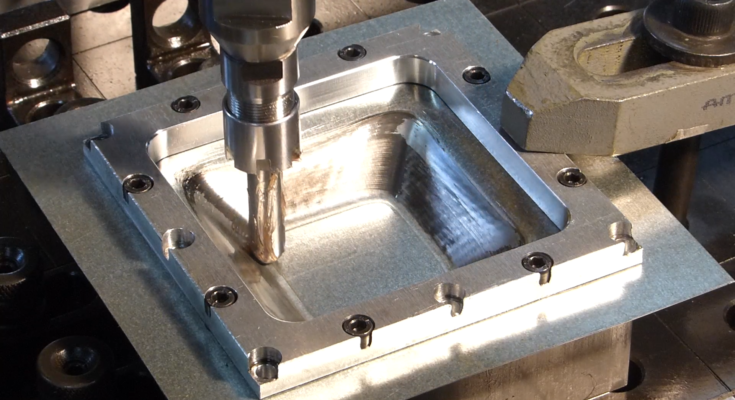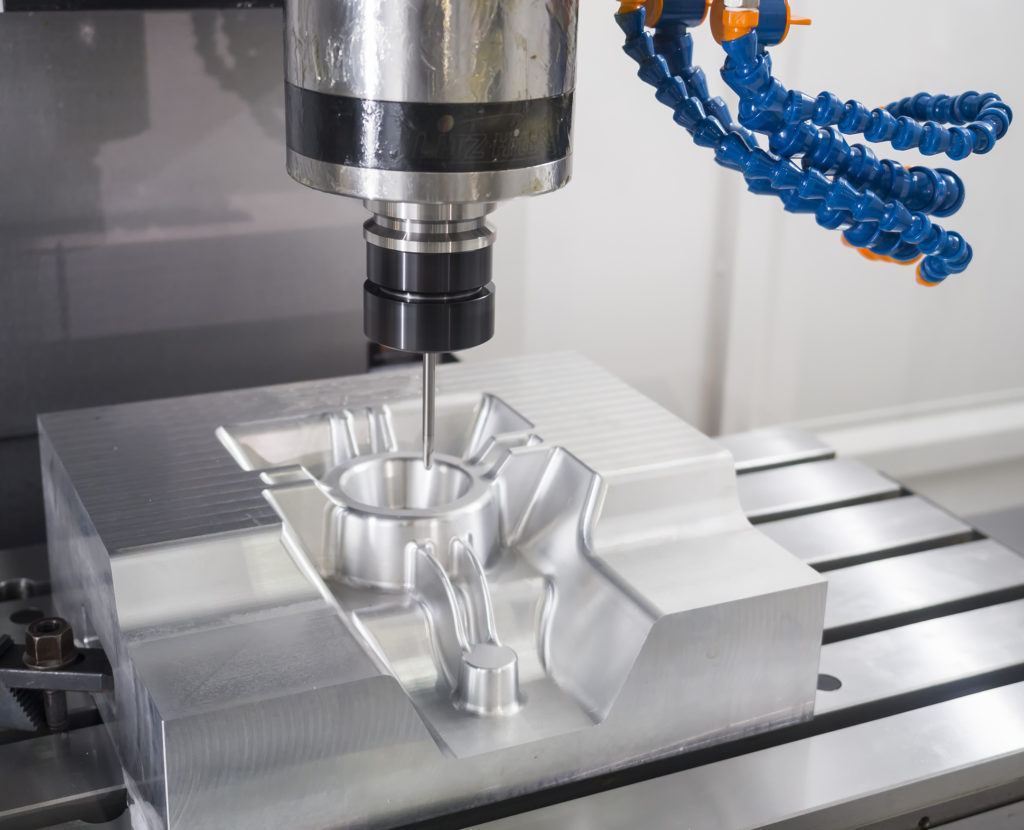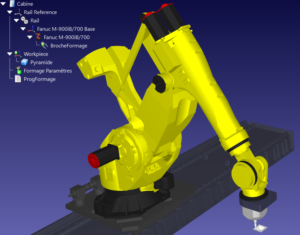# How to Save 90% Per Part With Robotic Incremental FormingWhy is sheet forming so expensive!? We explain why and show how to calculate how much you could save by using robotic incremental forming.

Forming sheet material can be expensive. Unless you’re manufacturing huge runs of identical parts the cost can be restrictive, particularly if you are a high-mix, low-volume manufacturer.

What are you supposed to do? Should you just give up on using sheet materials altogether? No.

Thankfully, there’s a way that you can get the benefits of sheet forming at a cost-effective price: robotic incremental forming. All you need is a robot and a copy of RoboDK.

In this article, we’ll explain why traditional sheet forming is so expensive and how you can calculate your potential savings.

## 5 Factors Which Make Traditional Sheet Forming ExpensiveOne of the most common methods of sheet material forming is deep drawing, which involves stretching material over a die.

One factor hugely affects the cost of this method:

1. Manufacturing the die — The main expense is the cost of manufacturing the die itself. This can easily be multiple times the cost of the production of each part. To balance out this cost, it is necessary to produce hundreds or thousands of the same part.

Other factors which drive up the cost of sheet forming are:

1. Costs associated with having many suppliers — Dies are often made by external die manufacturers. This is not ideal. According to research by consulting firm McKinsey, a major difference between prosperous and non-prosperous companies was that they limited their number of suppliers.
2. Long lead times for dies — Making a die takes a long time, particularly compared to the time for production. It can take days to design and manufacture a die. Incremental forming can be completed within minutes or hours (depending on the size and complexity of the part).
3. Scrap — Deep drawing produces more scrap. While scrap can be sold on to recoup some of the costs, it means that you must buy extra sheet material to account for it. For example, one study found that manufacturing a part using deep drawing required 12% more material than to manufacture the same part with incremental forming.
4. Lubricant — Deep drawing requires more and harsher lubricants than incremental forming does. This increases the cost in various ways, including requiring special airing systems, filters and protective clothes. It can also add up to 10% of the cost of the part to clean it afterwards.

Robotic incremental forming allows you to reduce these costs or, in some cases, eliminate them completely.

## 3 Steps to Calculate if Incremental Forming Will Save You Money

Okay, so robotic incremental forming can be more cost-effective. However, your question now is probably: Will it be cost-effective for me!?

There is are three simple steps to find out:

1. Calculate the cost of traditional sheet forming.
2. Calculate the cost of robotic incremental forming.
3. From your answers, calculate your break-even point. This will determine the batch size which will make the traditional process more cost-effective.

Let’s look at each of these steps one-by-one.

### 1. Calculate the Cost of Traditional Forming

The cost of traditional sheet forming can be split into two parts: cost of the die and cost of each part.

You can calculate the cost of any manufactured part using the following equation:

Use this equation to calculate both the cost of each formed sheet and the cost of the die. For manufacturing the die, the cycle time will be longer and the machining cost will be higher. The material cost may also be higher, depending on the materials used.

Total production cost is then:

As I mentioned above, deep drawing produces a reasonable amount of scrap, which will be added to the raw material cost.

#### Example

Using the example from this research study, let’s imagine that each deep drawn part requires 3.1 kg of material at a cost of $0.7/kg. It costs$25/hr to form each sheet and the deep drawing process can produce 420 parts per hour. Assume a 10% cost of scrap material and 100% efficiency.

Therefore the cost of a batch of 50 parts (excluding the cost of the die) is:

However, the cost of the die itself is found to be $3000, ten times larger than the cost per part. Therefore, the total production cost is$3122.15.

### 2. Calculate the Cost of Robotic Incremental Forming

The equation given above can also be used to calculate the “Cost per Part” of robotic incremental forming. The cycle time and hourly forming cost will be higher for incremental forming than it will for deep drawing.

The total cost is then:

As you can see, there is also a one-off fixture cost; however, this will be much lower than the cost to manufacture a die. There is no scrap cost but this can be included if applicable.

#### Example

Drawing from the same research study as before, let’s say that incremental forming of the same part requires 1.7 kg per part also at $0.7/kg. It costs$33/hr for the robotic forming process and it can produce 7.5 parts per hour. There is a one-off fixture cost of \$41.

For a batch of 50 parts, the cost is now:

As you can see, even though incremental forming takes a lot longer than deep drawing, the high cost of the die means that it is 90% cheaper to use incremental forming for this size of batch.

### 3. Calculate Your Break-even Point

How big does your batch need to be to justify deep drawing?

You can calculate your break-even point by using this combined equation, which compares the cost for traditional deep drawing and incremental forming:

For the example we have been using, this works out at a batch size of 726.13. Therefore, if the batch is 726 or less, incremental forming is more cost-effective. If the batch is 727 or more then traditional forming is better.

For small batches, you will often find that this is the case.

## How to Achieve Robotic Incremental Forming with RoboDK

It is surprisingly easy to use a robot for incremental forming. Using RoboDK, you can generate the robot code using standard G-Code in a matter of minutes using our Machining Tool.#### About Alex Owen-Hill

Alex Owen-Hill is a freelance writer and public speaker who blogs about a large range of topics, including science, presentation skills at CreateClarifyArticulate.com, storytelling and (of course) robotics. He completed a PhD in Telerobotics from Universidad Politecnica de Madrid as part of the PURESAFE project, in collaboration with CERN. As a recovering academic, he maintains a firm foot in the robotics world by blogging about industrial robotics.

View all posts by Alex Owen-Hill